##### Common Core Math For Parents For Dummies with Videos Online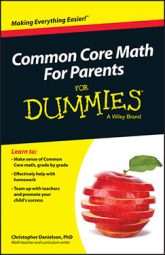Sixth grade is the first time that Common Core math students start to solve problems using variables and the property of equivalence (specifically, the distributive property).

They typically use variables that closely match the values they represent, so s for side length, v for volume, and so on. They also notice that some expressions — even though they may look quite different — always have the same values as each other, and they explore this property, called equivalence.

## Solve problems using variables

Sixth graders are introduced to variables (letters that stand for numbers) for the first time. Mostly, they're concrete applications of variables in which students represent simple numerical or measurement relationships.

An example of using variables to represent numerical relationships might be expressing the number of wheels on x cars as 4x or an unknown number of boys in a class as b, an unknown number of girls in a class as g, and the number of students in the class as b + g.

An example of using variables to represent measurement relationships is showing the perimeter of a square as 4s and the area of a square as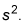In these two cases, s represents the side length of the square, and students show how to use the side length to compute the other measure — either perimeter or area.

Beginning the use of variables by expressing these relationships in familiar scenarios allows students to get a feeling for the way algebra shows the structure of a situation, whereas arithmetic only shows individual values.

## Solve problems using the property of equivalence

Students come to sixth grade knowing that there are many ways to write the same value. The number 459 can be written as 400 + 50 + 9, for example, or 460 – 1,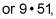and so on. Similarly, the fractions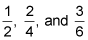all have the same value. Students are accustomed to calling fractions equivalent when they have the same value.

This idea of same value is extended in sixth grade to include equivalent expressions. Two equivalent expressions have the same value whenever their variable has the same value. The expressions 4(x + 2) and 4x + 8 have the same value as each other for all values of x, so they're equivalent. The expressions x + 2 and 2x only have the same value when x is equal to 2, so they aren't equivalent.

In sixth grade, students use simple examples of the distributive property to write and to justify that two expressions are equivalent. The distributive property states that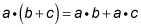If you think of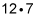as 70 + 14, then you're using the distributive property because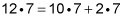The example of 4(x + 2) and 4x + 8 is an example of the distributive property.